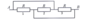# What is the total resistance?

• Kara386

## Homework Statement

In the circuit attached below, what's the resistance between A and B?

## The Attempt at a Solution

I'm finding it difficult to interpret this thing. Are resistors 1 and 2 in series or parallel? What does the loop above 1 and 2 actually do, in terms of how much current would flow round it? It has no components in it. I really have no idea what's going on in the diagram.

I'm guessing it involves Kirchhoff's current and voltage laws but I'm struggling to actually apply those here. Thanks for any help!

#### Attachments

•circuit2.png
2.9 KB · Views: 379
Last edited:

This exact problem has shown up here several times. You need to just pay attention to the definitions of serial and parallel connections and redraw the circuit. That is that POINT of the exercise, actually, more than the answer. It's to force you to pay close attention to the definitions.

•Kara386
This exact problem has shown up here several times. You need to just pay attention to the definitions of serial and parallel connections and redraw the circuit. That is that POINT of the exercise, actually, more than the answer. It's to force you to pay close attention to the definitions.
Are these just all in parallel? If I removed either of those loops I'd say what was left was three resistors in parallel, but what happens when there are two loops? Still just in parallel?

Are these just all in parallel? If I removed either of those loops I'd say what was left was three resistors in parallel, but what happens when there are two loops? Still just in parallel?
Yes, it's rather trivial to note that each of the resistors has one end hooked to the input and one to the output so they are obviously in parallel. You'll need to define what you mean by "two loops" to get an answer to that, but if you draw the diagram properly it will be obvious in any case.

•Kara386
Yes, it's rather trivial to note that each of the resistors has one end hooked to the input and one to the output so they are obviously in parallel. You'll need to define what you mean by "two loops" to get an answer to that, but if you draw the diagram properly it will be obvious in any case.
I see it, thank you! :)